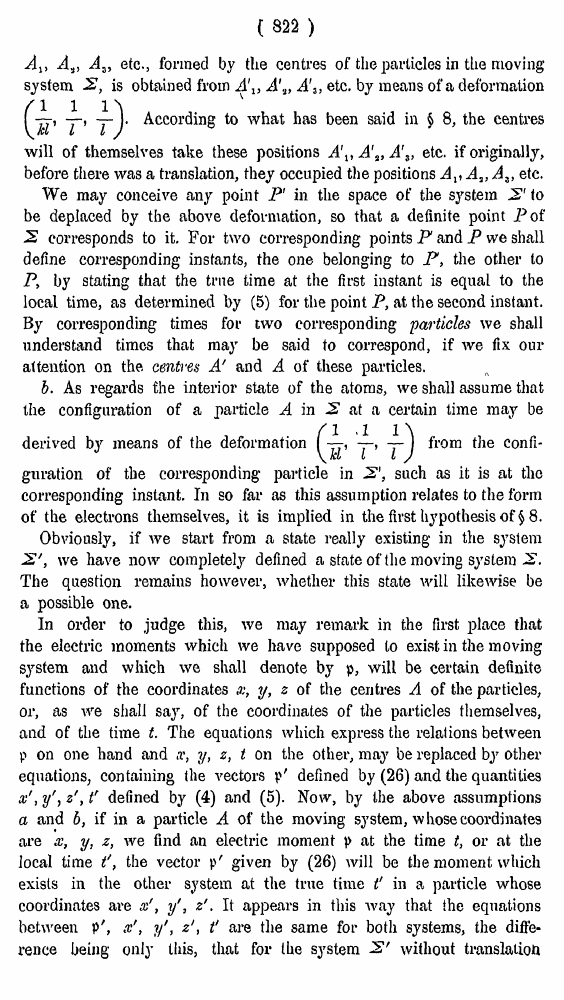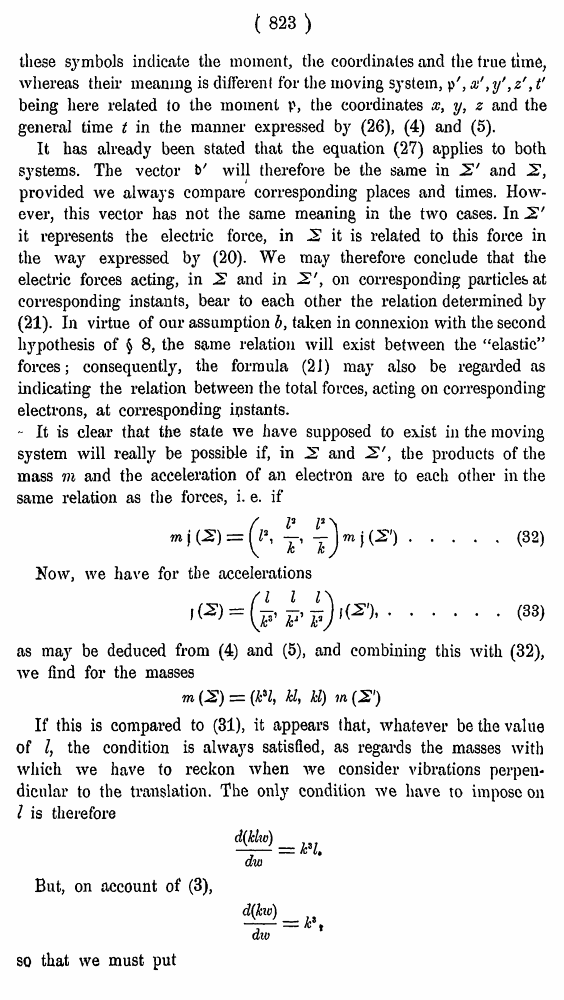# H. A. Lorentz. Electromagnetic phenomena in a system moving with any velocity smaller than that of light. // Proceedings Royal Acad., Amsterdam. Vol. VI., 1904

В начало   Другие форматы   <<<     Страница 822   >>>809  810  811  812  813  814  815  816  817  818  819  820  821  822 823  824  825  826  827  828  829  830  831 ( 822 ) A%, A.}, Az, etc., formed by the centres of the particles in the moving system 2, is obtained from A'1} A\, A'3, etc. by means of a deformation According to what has been said in § 8, the centres will of themselves take these positions A\t A's, A'3> etc. if originally, before there was a translation, they occupied the positions Av A,, At> etc. We may conceive any point P' in the space of the system 2' to be deplaced by the above deformation, so that a definite point Pof 2 corresponds to it. For two corresponding points P and P we shall define corresponding instants, the one belonging to P, the other to P, by stating that the true time at the first instant is equal to the local time, as determined by (5) for the point P, at the second instant. By corresponding times for two corresponding 'particles we shall understand times that may be said to correspond, if we fix our attention on the. centres A' and A of these particles. b. As regards the interior state of the atoms, we shall assume that the configuration of a particle A in 2 at a certain time may be derived by means of the deformationfrom the confi guration of the corresponding particle in 2', such as it is at tlio corresponding instant. In so far as this assumption relates to the form of the electrons themselves, it is implied in the first hypothesis of \$8. Obviously, if we start from a state really existing in the system 2', we have now completely defined a state of the moving system 2. The question remains however, whether this state will likewise be si possible one. In order to judge this, we may remark in the first place that the electric moments which we have supposed to exist in the moving system and which we shall denote by p, will be certain definite Functions of the coordinates x, y, z of the centres A of the particles, )r, as we shall say, of the coordinates of the particles themselves, xnd of the time t. The equations which express the relations between ? on one hand and x, ?/, z, t on the other, may be replaced by other jquations, containing the vectors p' defined by (26) and the quantities v', y'j z', t' defined by (4) and (5). Now, by the above assumptions i and b, if in a particle A of the moving system, whose coordinates ire x, y, z, we find an electric moment |> at the time t, or at the ocal time t', the vector p' given by (26) will be the moment which ixists in the other system at the true time t' in a particle whose loordinates are x', yf, z'. It appears in this way that the equations letween p', x', y', zt' are the same for both systems, the diffe* •ence being only this, that for the system 2' without translation ( 823 ) these symbols indicate the moment, the coordinates and the true time, whereas their meaning is different for the moving system, p', x' ,y' ,z' ,t' being here related to the moment p, the coordinates x, y, z and the general time t in the manner expressed by (26), (4) and (5). It has already been stated that the equation (27) applies to both systems. The vector b' will therefore be the same in 2' and 2, provided we always compare corresponding places and times. However, this vector has not the same meaning in the two cases. In 2' it represents the electric force, in 2 it is related to this force in the way expressed by (20). We may therefore conclude that the electric forces acting, in 2 and in 2', on corresponding particles at corresponding instants, bear to each other the relation determined by (21). In virtue of our assumption b, taken in connexion with the second hypothesis of § 8, the same relation will exist between the “elastic” forces; consequently, the formula (21) may also be regarded as indicating the relation between the total forces, acting on corresponding electrons, at corresponding ipstants. - It is clear that the state we have supposed to exist in the moving system will really be possible if, in 2 and 2', the products of the mass Til and the acceleration of an electron are to each other in the same relation as the forces, i. e. if .....(32) Now, we have for the accelerations ......(33) as may be deduced from (4) and (5), and combining this with (32), we find for the masses If this is compared to (31), it appears that, whatever be the value of I, the condition is always satisfied, as regards the masses with which we have to reckon when we consider vibrations perpendicular to the translation. The only condition we have to impose on I is therefore But, on account of (3), so that we must put